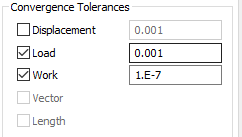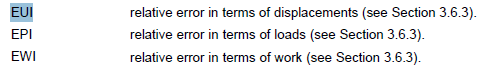# Monitoring nonlinear solutions with the Femap Analysis Monitor

## Monitoring nonlinear solutions with the Femap Analysis Monitor

An explanation is required to understand what Femap’s Analysis Monitor is telling you for SOL106: Basic Nonlinear solutions.  Specifically, the “Load Step Convergence” option shown below.  Note the circled quantities.  They’ll be part of the explanation for this example.The convergence ratio is showing the trend of each iteration’s convergence factor (e.g. EPI) divided by the tolerance on NLPARM card (e.g. EPSP).  Here’s the last iteration’s info from the F06 on this quick test FEM:

Note that at the final step, EPI = 5.4e-4 in the f06 file.  Femap’s analysis monitor shows 0.54, which is = EPI / EPSP  = 5.4e-4 / 1e-3.  When you set up NL, you specify these convergence tolerances:In Nastran speak, these values are:The method for calculating these relative error values are found in section 3.6.3 of the NL theoretical handbook.  (Typical location – “C:\FEMAPv1131\nastranhelp\pdf\nonlinear_106_NXN.pdf”).  There you can find the calculation of EUI, EPI, EWI.  EPI, for example, is calculated as follows:Is this sort of data used in the real world engineering?  Sometimes convergence tolerances are changed, but this is not the recommended goto fix for analyses that fail to converge.  It is highly likely that either another aspect of model setup or the modeled physical behavior is at fault for lack of convergence.

If you actually want to monitor convergence, don’t use this F06 file data.  Think of the F06 data as the low-level math behind your solution.  It is far easier to understand the high-level output data.  Track the actual output in the region of interest instead.  For example, monitor strain output as a function of loading near a stress/ strain concentration point.  The loading profile can come from intermediate output that you request with nonlinear setup options.  Be sure to request this in your analysis nonlinear options:Then, use Femap’s charting feature to plot the output versus output set.  Each output set is the loading profile mentioned earlier.